Courses

# Important Definitions & Formulas: Pair of Linear Equations in Two Variables Class 10 Notes | EduRev

## Class 10 : Important Definitions & Formulas: Pair of Linear Equations in Two Variables Class 10 Notes | EduRev

The document Important Definitions & Formulas: Pair of Linear Equations in Two Variables Class 10 Notes | EduRev is a part of the Class 10 Course Mathematics (Maths) Class 10.
All you need of Class 10 at this link: Class 10

Equation

An equation is a mathematical statement that two mathematical expressions having one or more variables are equal.

Linear Equation in Two Variables

The word 'Linear' means single degree equation i.e. the maximum powers of all the variables involved are one. The word 'Two Variables' means that the mathematical statement will be having two variables i.e. two mathematically unknown quantities.

The general form of a linear equation in two variables is ax+by+c=0, where a and b cannot be zero simultaneously.

Example: 2x+4y-8=0

Pair of Linear Equations in Two VariablesThe general form of a pair of linear equations in two variables x and y is
a1x + b1y + c1 = 0, and

a2x + b2y + c2 = 0
where a1, b1, c1, a2, b2, c2 are all real numbers and the coefficients of x and y cannot be zero simultaneously.

Solution of Pair of Linear Equations in Two Variables

A pair of values of x and y, satisfying each one of the equations, is called a solution of the linear equations in two variables.
A pair of linear equations in two variables can be represented and solved by:
1. Graphical Method
2. Algebraic Methods

1. Graphical Method for Solving a Pair of Linear Equations in Two Variables

We draw the graph of two equations in x and y on the same graph :
(a) If the two lines intersect, then the equations are consistent and a unique solution is given by the coordinates of the point of intersection.
(b) If the two lines are parallel, then the equations are inconsistent and they have no solution.
(c) If the two lines coincide each other, then the equations are consistent and they have an infinite number of solutions. Such equations are called dependent equations.

2. Algebraic Methods for Solving a Pair of Linear Equations in Two Variables

We have three algebraic methods of solution for a pair of linear equations in two variables:

(a) Substitution Method

Step 1: Find the value of one variable, say y in terms of the other variable, i.e., x from either equation, whichever is convenient

Step 2: Substitute this value of y in the other equation, and reduce it to an equation in one variable, i.e., in terms of x, which can be solved. Sometimes, you will get statements with no variables. If this statement is true, you can conclude that the pair of equations has infinitely many solutions. If the statement is false, then the pair of linear equations is inconsistent.

Step 3: Substitute the value of x (or y) obtained in Step 2 in the equation used in Step 1 to obtain the value of the other variable.

(b) Elimination Method or Method of Elimination by Equating the Coefficients
Step 1: First, multiply both the equations by some suitable non-zero constants to make the coefficients of one variable (either x or y) numerically equal.

Step 2: Then add or subtract one equation from the other so that one variable gets eliminated. After you get an equation in one variable, go to step 3.

If in Step 2, you obtain a true statement involving no variable, then the original pair of equations has infinitely many solutions.

If in Step 2, you obtain a false statement involving no variable, then the original pair of equations has no solution, i.e., it is inconsistent.

Step 3: Solve the equation in one variable(x or y) so obtained to get its value.

Step 4: Substitute this value of x ( or y) in either of the original equations to get the value of the other variable.

(c) Cross-Multiplication Method
Step 1: Write both of the given equations with their variables and constant term to the left-hand side.
Step 2: Write the coefficients of x,y and the constant term as: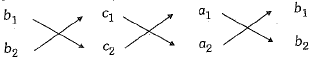Step 3: Now, obtain three equal fractions with numerators x, y and 1, for their denominators, multiply the numbers with downward arrows and from their product subtract the product of the numbers with upward arrows.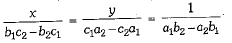Step 4: Now, obtain the value of x by equating the first and last term and the value of y by equating the second and last term. The values of x and y obtained above are the solution of the given equations.

Conditions for Consistency/Inconsistency

A pair of linear equations in two variables, which has a solution, is called consistent and a pair of linear equations in two variables, which has no solution is called inconsistent. If a pair of linear equations in two variables is given b
a1x + b1y + c1 = 0 and a2x + b2y + c2 = 0, then they have
(i) unique solution (consistent),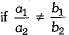(ii) infinite number of solutions (consistent), if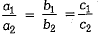NOTE: These equations are also known as coincident lines or dependent lines.

(iii) no solution (inconsistent),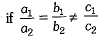Equations Reducible to a Pair of Linear Equations in Two Variables

There are several situations that can be mathematically represented by two equations that are not linear but can be reduced to linear by making certain suitable substitutions.

Word Problems

Read the statement carefully and identify the unknown quantities. Represent the unknown quantity by x, y, z, a, b, c, etc. Formulate the equations in terms of the variables to be determined and solve the equations to get the values of the required variables. Finally, verify with the conditions of the original problem. The problems are stated in words, for this reason, often refers to word problems.

Offer running on EduRev: Apply code STAYHOME200 to get INR 200 off on our premium plan EduRev Infinity!

## Mathematics (Maths) Class 10

51 videos|346 docs|103 tests

,

,

,

,

,

,

,

,

,

,

,

,

,

,

,

,

,

,

,

,

,

;